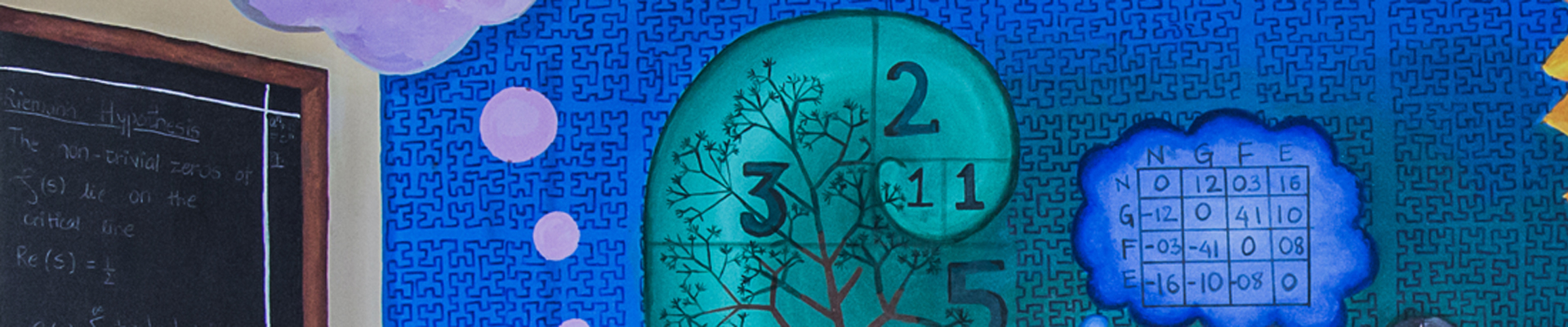# Department of Mathematics

### Mousomi Bhakta

Associate Professor; and Rahul Bajaj Chair Professor

Mathematics

Elliptic PDE, nonlinear analysis, variational methods

+91-20-25908253

mousomi@iiserpune.ac.in

Mousomi Bhakta obtained her PhD from TIFR-CAM in 2011 and then joined as a visiting scientist in ICTP, Italy for three months. She was a postdoc in Technion, Israel for 2 years and University of New England, Australia for a year before joining as an Assistant Professor at IISER Pune in 2014.

## Research

### Nonlinear Analysis, Elliptic PDE, Hardy potential, Equations with measure data

Dr. Mousomi Bhakta is studying the existence, multiplicity of positive and sign-changing solutions to local and nonlocal elliptic equations applying tools from nonlinear analysis and to study various qualitative properties of solutions e.g. radial symmetry, regularity, apriori estimate, etc. Dr. Bhakta studied the above questions to various local and nonlocal type semilinear and quasilinear elliptic equations with Hardy potential, equations with Hardy-Sobolev-Maz'ya type nonlinearities either in bounded domain or in the entire Euclidean space.

Another important topic of Dr. Bhakta's research is the study of local and nonlocal type singular elliptic system of equations with measure data. These type of solutions are called very weak solutions. Here too, her interest is to study existence, multiplicity, regularity of positive solutions.

## Selected Publications

Bhakta, M.; Chakraborty, S; Miyagaki O.H. and Pucci, P. (2022). Fractional elliptic systems with critical nonlinearities. to appear in Nonlinearity.

Bhakta, M.; Mukherjee, D. and Nguyen, P. T. (2022). Multiplicity and uniqueness for Lane-Emden equations and systems with Hardy potential and measure data. to appear in J. Differential Equations.

Bhakta, M.; Chakraborty, S.; Pucci, P. (2021). Fractional Hardy-Sobolev equations with nonhomogeneous terms. Adv. Nonlinear Anal. Vol 10, 1086–1116.

Bhakta, M.; Nguyen, P. T. (2020). On the existence and multiplicity of solutions to fractional Lane-Emden elliptic systems involving measures. Adv. Nonlinear Anal. Vol 9, 1480–1503.

Bhakta, M. and Mukherjee, D. (2019). Nonlocal scalar field equations: qualitative properties, asymptotic profiles and local uniqueness of solutions. J. Differential Equations. Vol 266, 6985–7037.

Bhakta, M. and Mukherjee, D. (2019). Multiplicity results for (p,q) fractional elliptic equations involving critical nonlinearities. Adv. Differential Equations. Vol 24, 185–228.

Bhakta, M.; Santra, S. (2017). On singular equations with critical and supercritical exponents. J. Differential Equations. Vol 263, 2886–2953.

Bhakta, M. and Marcus. M. (2014). Semilinear elliptic equations admitting similarity transformations. J. Funct. Anal. Vol 267, 3894–3930.

Bhakta, M. and Musina, R. (2012). Entire solutions for a class of variational problems involving the biharmonic operator and Rellich potentials. Nonlinear Anal. Vol 75, 3836–3848.

Bhakta, M. and Sandeep, K. (2012). Poincaré-Sobolev equations in the hyperbolic space. Calc. Var. Partial Differential Equations. Vol 44, 247–269.